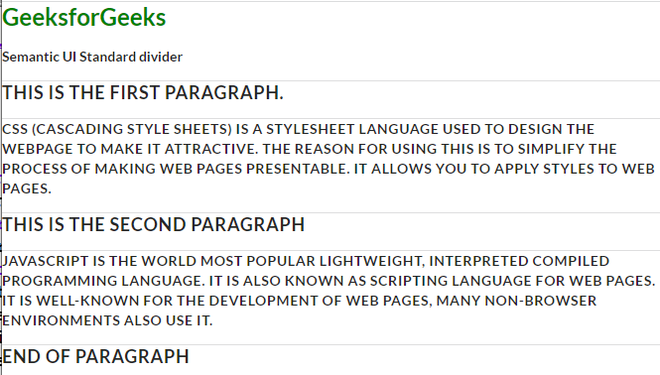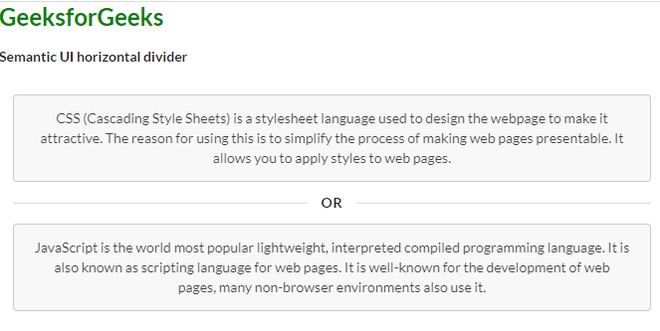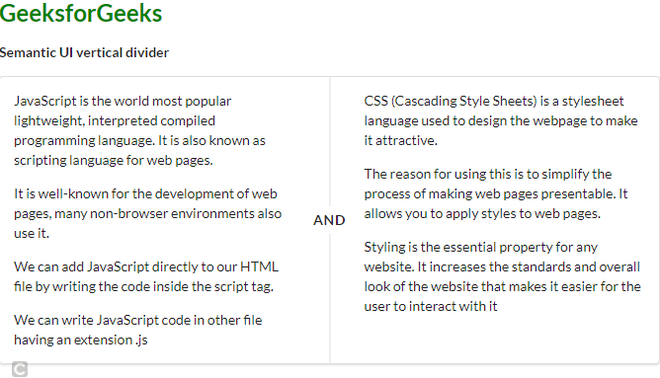Open in App
Not now

# Semantic-UI Divider Types

• Last Updated : 22 Apr, 2022

Semantic UI is a free open-source front-end development framework that is equipped with pre-built semantic components that helps create responsive layouts using user-friendly HTML. In terms of user-friendliness, Semantic UI is better than Bootstrap styling. Top companies like Snapchat, Accenture have started adopting Semantic UI for the frontend.

In this article, let us discuss different divider types that are available in Semantic UI. A divider is generally used to divide the content concisely into different sections.

Semantic-UI Divider Types Class:

• Divider: A conventional way of dividing the webpage content into different parts of a grid.
• Horizontal Divider: A divider can segment webpage content horizontally.
• Vertical Divider: A divider can segment webpage content vertically.

Syntax:

```<div class="ui horizontal divider">
....
</div>```

Example 1: The following example demonstrates the standard divider class.

## HTML

 `` `<``html``>`   `<``head``>` `    ``<``link` `href``=` `"https://cdnjs.cloudflare.com/ajax/libs/semantic-ui/2.4.1/semantic.min.css"` `        ``rel``=``"stylesheet"` `/>` ``   `<``body``>` `    ``<``h2` `style``=``"color:green"``>` `        ``GeeksforGeeks` `    ``` `    `  `    ``<``strong``>Semantic UI Standard divider` `    `  `    ``<``div` `class``=``"ui divider"``>` `        ``<``h3``> This is the first paragraph.` `        ``<``div` `class``=``"ui divider"``>` `            `  `<``p``>` `                ``CSS (Cascading Style Sheets) is a ` `                ``stylesheet language used to design ` `                ``the webpage to make it attractive.` `                ``The reason for using this is to ` `                ``simplify the process of making ` `                ``web pages presentable. It allows ` `                ``you to apply styles to web pages.` `            ```     `            ``<``div` `class``=``"ui divider"``>` `                ``<``h3``> This is the second paragraph` `                ``<``div` `class``=``"ui divider"``>` `                    `  `<``p``>` `                        ``JavaScript is the world most ` `                        ``popular lightweight, interpreted ` `                        ``compiled programming language.` `                        ``It is also known as scripting ` `                        ``language for web pages. It is ` `                        ``well-known for the development ` `                        ``of web pages, many non-browser ` `                        ``environments also use it.` `                    ```     `                    ``<``div` `class``=``"ui divider"``>` `                        ``<``h3``> End of paragraph ` `                    ``` `                ``` `            ``` `        ``` `    ``` ``   ``

Output:Semantic-UI Divider Types

Example 2: The following example will divide the section into two equal halves horizontally as shown below using the horizontal class.

## HTML

 `` `<``html``>`   `<``head``>` `    ``<``link` `href``=` `"https://cdnjs.cloudflare.com/ajax/libs/semantic-ui/2.4.1/semantic.min.css"` `        ``rel``=``"stylesheet"` `/>` ``   `<``body``>` `    ``<``h2` `style``=``"color:green"``>` `        ``GeeksforGeeks` `    ``` `    `  `    ``<``b``>` `        `  `<``p``>Semantic UI horizontal divider`   `    ``` `    `  `    ``<``div` `class``=``"ui center aligned basic segment"``>` `        ``<``div` `class``=``"ui message"``>` `            `  `<``p``>` `                ``CSS (Cascading Style Sheets) is ` `                ``a stylesheet\language used to ` `                ``design the webpage to make it` `                ``attractive. The reason for using ` `                ``this is to simplify the process ` `                ``of making web pages presentable. ` `                ``It allows you to apply styles to` `                ``web pages.` `            ```   `        ```   `        ``<``div` `class``=``"ui horizontal divider"``>` `            ``Or` `        ``` `        `  `        ``<``div` `class``=``"ui message"``>` `            `  `<``p``>` `                ``JavaScript is the world most ` `                ``popular lightweight, interpreted ` `                ``compiled programming language.` `                ``It is also known as scripting ` `                ``language for web pages. It is ` `                ``well-known for the development ` `                ``of web pages, many non-browser ` `                ``environments also use it.` `            ```   `        ``` `    ``` ``   ``

Output:Semantic-UI Divider Types

Example 3. This example will divide the section or columns into two equal halves vertically using the vertical class.

## HTML

 `` `<``html``>`   `<``head``>` `    ``<``link` `href``=` `"https://cdnjs.cloudflare.com/ajax/libs/semantic-ui/2.4.1/semantic.min.css"` `        ``rel``=``"stylesheet"` `/>` ``   `<``body``>` `    ``<``h2` `style``=``"color:green"``>` `        ``GeeksforGeeks` `    ``` `    `  `    ``<``b``>` `        `  `<``p``>Semantic UI vertical divider`   `    ``` `    `  `    ``<``div` `class``=``"ui segment"``>` `        ``<``div` `class``=``"ui two column very relaxed grid"``>` `            ``<``div` `class``=``"column"``>` `                `  `<``p``>` `                    ``JavaScript is the world most popular` `                    ``lightweight, interpreted compiled` `                    ``programming language. It is also ` `                    ``known as scripting language for web ` `                    ``pages.` `                ```     `                `  `<``p``>` `                    ``It is well-known for the development ` `                    ``of web pages, many non-browser ` `                    ``environments also use it.` `                ```     `                `  `<``p``>` `                    ``We can add JavaScript directly to ` `                    ``our HTML file by writing the code ` `                    ``inside the script tag.` `                ```     `                `  `<``p``>` `                    ``We can write JavaScript code in ` `                    ``other file having an extension .js` `                ```     `            ``` `            ``<``div` `class``=``"column"``>` `                `  `<``p``>` `                    ``CSS (Cascading Style Sheets) is a ` `                    ``stylesheet language used to design ` `                    ``the webpage to make it attractive.` `                ```     `                `  `<``p``>` `                    ``The reason for using this is to ` `                    ``simplify the process of making ` `                    ``web pages presentable. It allows ` `                    ``you to apply styles to web pages.` `                ```     `                `  `<``p``>` `                    ``Styling is the essential property ` `                    ``for any website. It increases the ` `                    ``standards and overall look of the ` `                    ``website that makes it easier for ` `                    ``the user to interact with it` `                ```   `            ``` `        ```   `        ``<``div` `class``=``"ui vertical divider"``>` `            ``and` `        ``` `    ``` ``   ``

Output:Semantic-UI Divider Types

My Personal Notes arrow_drop_up
Related Articles